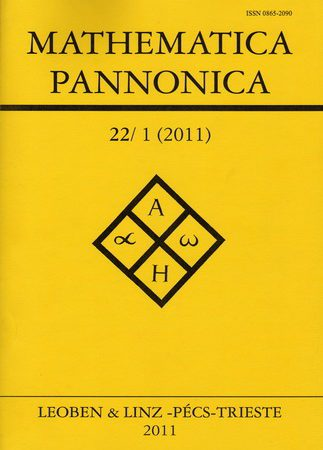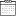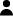# Harvest management problem with a fractional logistic equation

• Home
• Harvest management problem with a fractional logistic equation6 September 2021By CEE-M

2021In this article, we study a fractional control problem that models the maximization of the pro fit obtained by exploiting acertain resource whose dynamics are governed by the fractional logistic equation. Due to the singularity of this problem, wedevelop di fferent resolution techniques, both for the classical case and for the fractional case. We perform several numerical simulations to make a comparison between both cases.